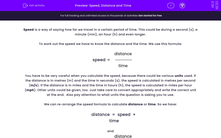# Relate Speed, Distance and Time

In this worksheet, students will practise calculating speed and what affects it.Key stage:  KS 3

Curriculum topic:   Physics: Motion and Forces

Curriculum subtopic:   Describing Motion

Popular topics:   Physics worksheets

Difficulty level:#### Worksheet Overview

Speed is a way of saying how far we travel in a certain period of time. This could be during a second (s), a minute (min), an hour (h) and even longer.

To work out the speed we have to know the distance and the time. We use this formula:

 distance speed = time

You have to be very careful when you calculate the speed, because there could be various units used. If the distance is in metres (m) and the time in seconds (s), the speed is calculated in metres per second (m/s). If the distance is in miles and the time in hours (h), the speed is calculated in miles per hour (mph). Other units could be given, too. Just take care to convert appropriately and write the correct unit at the end.  Also pay attention to what units the question is asking you to use.

We can re-arrange the speed formula to calculate distance or time. So we have:

 distance  =  speed  ×  time

and

 distance time = speed

When drivers see something on the road, it takes them some time to brake. The distance travelled during this time is called thinking distance. After braking, the car travels a certain distance before it finally stops; this is called braking distance. The total of those two is the stopping distance. The stopping distance depends on the initial speed of the car and the alertness of the driver.

Below you can see a distance-time graph. Study the different parts of the graph carefully.Key points:

- A straight diagonal line means the object is moving at a constant speed.

- The steeper the line, the faster the speed.

- A flat line means the object is not moving.

- A downwards slope means the object is moving in the opposite direction.

### What is EdPlace?

We're your National Curriculum aligned online education content provider helping each child succeed in English, maths and science from year 1 to GCSE. With an EdPlace account you’ll be able to track and measure progress, helping each child achieve their best. We build confidence and attainment by personalising each child’s learning at a level that suits them.

Get started#### Popular Science topics

••••